## Pascal's Triangle

A Triangle of numbers arranged in staggered rows such that(1)

whereis a Binomial Coefficient. The triangle was studied by B. Pascal,although it had been described centuries earlier by Chinese mathematician Yanghui (about 500 years earlier, in fact) and the Arabian poet-mathematician Omar Khayyám. It is therefore known as the Yanghui Triangle in China. Starting with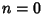, the Triangle is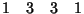(Sloane's A007318). Pascal's Formula shows that each subsequent row is obtained by adding the two entries diagonally above,(2)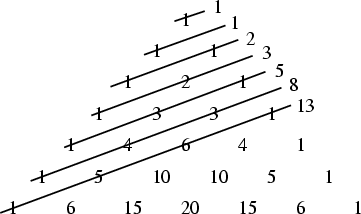In addition, the Shallow Diagonals'' of Pascal's triangle sum to Fibonacci Numbers,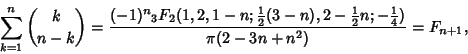(3)

whereis a Generalized Hypergeometric Function.

Pascal's triangle contains the Figurate Numbers along its diagonals. It can be shown that(4)

and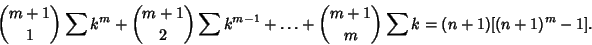(5)

The shallow diagonals'' sum to the Fibonacci Sequence, i.e.,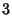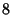(6)(7)

It is also true that the first number after the 1 in each row divides all other numbers in that row Iff it is a Prime. If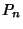is the number of Odd terms in the firstrows of the Pascal triangle, then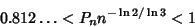(8)

(Harborth 1976, Le Lionnais 1983).

The Binomial Coefficientmod 2 can be computed using the XOR operationXOR, making Pascal's triangle mod 2 very easy to construct. Pascal's triangle is unexpectedly connected with the construction of regular Polygons and with the Sierpinski Sieve.

See also Bell Triangle, Binomial Coefficient, Binomial Theorem, Brianchon's Theorem, Catalan's Triangle, Clark's Triangle, Euler's Triangle, Fibonacci Number, Figurate Number Triangle, Leibniz Harmonic Triangle, Number Triangle, Pascal's Formula, Polygon, Seidel-Entringer-Arnold Triangle, Sierpinski Sieve, Trinomial Triangle

References

Conway, J. H. and Guy, R. K. Pascal's Triangle.'' In The Book of Numbers. New York: Springer-Verlag, pp. 68-70, 1996.

Courant, R. and Robbins, H. What is Mathematics?: An Elementary Approach to Ideas and Methods, 2nd ed. Oxford, England: Oxford University Press, p. 17, 1996.

Harborth, H. Number of Odd Binomial Coefficients.'' Not. Amer. Math. Soc. 23, 4, 1976.

Le Lionnais, F. Les nombres remarquables. Paris: Hermann, p. 31, 1983.

Pappas, T. Pascal's Triangle, the Fibonacci Sequence & Binomial Formula,'' Chinese Triangle,'' and Probability and Pascal's Triangle.'' The Joy of Mathematics. San Carlos, CA: Wide World Publ./Tetra, pp. 40-41 88, and 184-186, 1989.

Sloane, N. J. A. Sequence A007318/M0082 in An On-Line Version of the Encyclopedia of Integer Sequences.'' http://www.research.att.com/~njas/sequences/eisonline.html and Sloane, N. J. A. and Plouffe, S. The Encyclopedia of Integer Sequences. San Diego: Academic Press, 1995.

Smith, D. E. A Source Book in Mathematics. New York: Dover, p. 86, 1984.